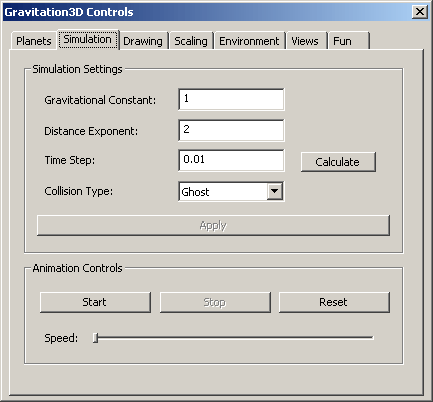The Simulation TabThis page gives you control over the simulation.  “Simulation Settings” include items that directly affect how the calculation engine will determine the orbits.  The “Animation Controls” options start, stop, and reset the simulation.

Simulation Settings

Reference Newton’s equation for the gravitational force between 2 bodies.

F = G * m1 * m2 / d ^ 2

 Gravitational Constant G” in the equation.  The default is given as 1.0 for convenience. Distance Exponent “2” in the equation. Time Step The time (in seconds) between each successive iteration in the simulation.  The default is 0.01 seconds (a value I have found to be a good starting point when G = 1).  1 day (86400 seconds) is a good starting point for true MKS units.  A lower time step is more accurate, but will slow your simulation and may be unnecessary.  A larger time step can easily become unstable. To help you find a good starting point, there is a button to calculate a default time step.  This calculation makes a best guess at a time step using the value you have for G and certain assumptions about the nature of typical systems.  It is usually enough to get you in the right ball park. Collision Type Elastic - Planets bounce off each other, acting like billiard balls. Inelastic - Planets stick together, acting like silly putty. Ghost (Default) - No collisions.  If planets intersect, they will simply move through each other.  The force between planets when intersected is zero however.  This is to reduce the instability that comes with planet centers that come very close to each other.  Note that the theoretical force between 2 planets at the same location is infinite because the distance between them is zero.

Animation Controls

Though the ‘A’ and ‘R’ keys also control starting, stopping, and resetting the simulation, extra buttons are provided here for convenience.

The animation speed is also controllable via a slider.

Once you have found a stable time step, it is preferable to use the animation speed slider to control the apparent speed of your simulation.  Unlike the time step, this slider does not affect the accuracy of the simulation.